# Computer help - file server

[ Blog ] - [ File Server ] - [ Удаленная компьютерная помощь ]

Excel is such a powerful spreadsheet program that I doubt anyone can possibly know everything that it can do. There are so many aspects to the program, it`s amazing that it can all be encapsulated in such a simple interface. I was recently using Excel for some work at home and found myself using the IF function to write a few formulas.

Being able to write a simple IF statement in Excel is essential, especially if you deal with a lot of numbers all the time. It`s one of those things that`s not too complicated like macros, but not necessarily intuitive like summing up a column of numbers. IF Statements in Excel

In this article, I`ll walk you through a short primer on writing a IF statement in an Excel worksheet. To get started, you should understand the basis of all IF statements: IF condition THEN true ELSE false

That`s pretty much it. The condition is usually a comparison of something. That something is normally numbers or text. We`ll start off with some basic number comparisons, then text, then include operators like AND/OR, etc. So here`s our first example: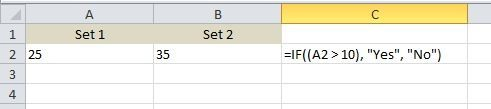We have a very simple example here. Two columns with two numbers, 25 and 35. If Column C, we want to check if Column A is greater than 10, we will output the text Yes, otherwise the text No. A few things to note:

1. You don`t have to put a set of parenthesis around the actual comparison, but it`s very good practice, especially when the comparison becomes more complicated.

2. To output text for the true or false value, you have to use double quotes. If you don`t, it will think you are trying to refer to a named range in Excel.

Now let`s see some more stuff you can do. In the comparison section, you can also do math too. For example, you can do this if you like: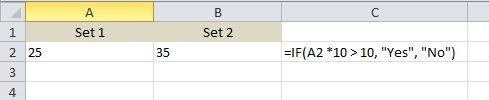Here we are saying that if the value of A2 times 10 is greater than 10, then output Yes, otherwise output No. Pretty straight-forward eh? Now let`s say we wanted to output numbers instead of text. Here`s one example: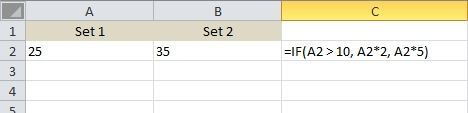In this example, if the value in A2 is greater than 10, it will output the value times 2, otherwise it will multiply the value by 5. Pretty useless, but you get the point. You can also use the value of other cells too in the formula.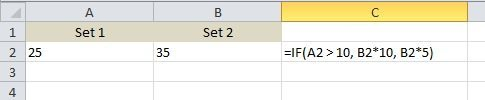Here we are saying that if the value of A2 is greater than 10, then multiple B2 by 10, otherwise multiple B2 by 5 and output that value into cell C2. Now let`s make it more complicated. What if we wanted to output Yes if both A2 and B2 are greater than 10?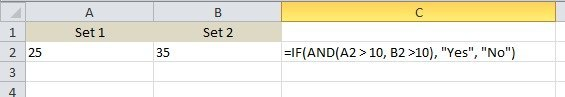Here`s something new! If we want A2 > 10 AND B2 > 10, then we have to use the AND function inside the IF function. Sounds tricky, but not too bad. The AND function basically takes multiple comparison arguments (A2 > 10) and does an AND on them. They all have to be true in order for “Yes” to be displayed. You can add three or four or more comparison arguments if you like. If you want to do an OR, simply replace the AND above with the word OR.

What about if you want to return a value other than text or a calculated number. What if you want to return the value of a cell on a different sheet? Well, you can do something like this: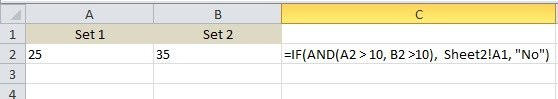For the true value, I am going to return the value of cell A1 on Sheet 2. That`s pretty easy! So you can easily reference values from other sheets if you like. In the same light, you can also use a value from another sheet in the logical comparison too, i.e. Sheet2!A1 > 10. Let`s look at more stuff you can do! Let`s say you wanted to do an IF statement based on the type of value stored in a cell (number, text, or blank). You can do something like this: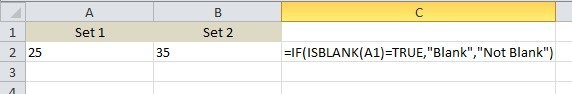You can use ISTEXT and ISNUMBER for the other two types of data. These functions can be really convenient when writing IF formulas. Note that you can basically use most functions in Excel inside of a IF statement, so feel free to run loose. For example, you can use functions like SUM, MAX, MIN, etc.

So far we`ve only been working with one value in the logical comparison, but let`s say we want to work with a group of cells. For example, if all the numbers in a range of cells is greater than 10, output True, otherwise False.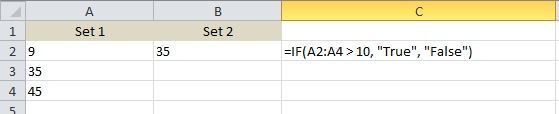It`s as simple as using the colon like shown above, A2:A4. In this case, each value from A2 to A4 will be tested to see if it`s greater than 10. If all of them are, then the true value will be displayed. What about nested IF statements?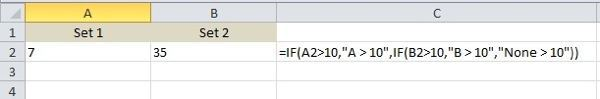Here, I am saying that if A2 is greater than 10, output a value, but if not, then perform another IF comparison. IF B2 is greater than 10, then output a different value. You can nest IF statements 64 levels deep, which means you can do some pretty complicated stuff. You`ll probably never need to go that far, but sometimes it`s useful a few levels deep.

This is only a basic introduction to the IF statement, but hopefully it`ll get you started. Are you trying to write an IF statement to do something in Excel and can`t figure it out? Post a comment here with the problem and I`ll try to help you write the proper IF formula. Enjoy!

SETUP UA COMPUTER BLOG